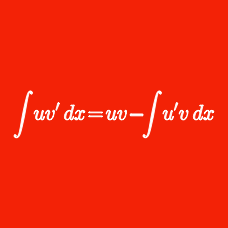Calculus

# Integration by Parts

Find the indefinite integral $$\displaystyle{ 43\int{x \ln x dx} },$$ using $$C$$ as the constant of integration.

Evaluate the integral $$\displaystyle{ 128\int{x \cos 3x dx} },$$ using $$C$$ as the constant of integration.

Find the indefinite integral $$\displaystyle{ \int{x \sqrt{x+19} dx} },$$ using $$C$$ as the constant of integration.

Evaluate the integral $$\displaystyle{ 67\int{t^2 e^{3t} dt} },$$ using $$C$$ as the constant of integration.

Evaluate the integral $$\displaystyle{ 43\int _{ 0 }^{ 1 }{ \arctan x dx } },$$ using $$C$$ as the constant of integration.

×